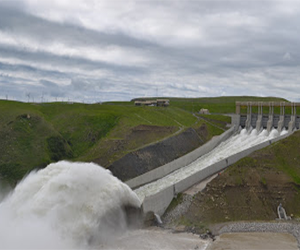# How to Calculate Reynold’s NumberThe Reynolds number of a fluid is a dimensionless constant which allows you to determine whether the flow of a fluid is laminar or turbulent. It represents the ratio of inertial forces to viscous forces in the fluid.$R_e = \frac{inertial forces}{viscous forces}$

The equation is:$R_e = \frac{D_ev}{\upsilon}$

Where:
De = Hydraulic Diameter (ft or m)
v = velocity of the fluid (ft/s or m/s)
υ = kinematic viscosity of the fluid (ft2/s or m2/s)

The Reynolds number allows one to determine whether the flow is laminar, turbulent, or within the transition zone between the two.

• Laminar: Less than 2100
• Transition: 2100 to 4000
• Turbulent: Greater than 4000

Laminar flow is flow in a straight line, i.e. If all of the particles are moving parallel, it is perfectly laminar.

## If the absolute viscosity is known

If you know the absolute, instead of the kinematic, viscosity of the fluid, you can revise the Reynolds equation to accomodate:$R_e = \frac{D_ev\rho}{\upsilon \mu}$

This is a simple conversion from the kinematic viscosity equation:$\upsilon = \frac{\mu}{\rho}$

## If the mass flow rate is known

Sometimes you might know the mass flow rate per unit area, G = ρv. In this case, the Reynolds number equation can also be modified to accomodate:$R_e = \frac{D_eG}{\mu}$

This site uses Akismet to reduce spam. Learn how your comment data is processed.